General Question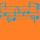Is there a general way to solve equations involving both a multiple of x and a power of x?

Asked by dxs (15160) March 28th, 2016

Say I’m trying to solve 2^x – 10 = 20 for x. I want to do it using algebra. Is this possible? If not, can I use calculus somehow? I’m looking for a way to solve it other than qualitatively (i.e., looking at a graph).

==========================

This problem arose when I was trying to find the point at which two graphs intersect. They were f(x) = 2^(x-1)/100 + 0.005 and g(x) = 5x + 10. The problem is for a middle school lesson I’m teaching. Finding their intersection algebraically was something I wanted to do with them since they’re an advanced class, but now I realize even I can’t do it. The problem is about a kid who earns \$10 a day, plus \$5 for every chore he does. On the first day of the month, he does one chore, on the 2nd, he does 2, and so on. He hates doing dishes, so his friend offers to do the dishes for him for a “bargain” price. He charges 1 cent on the first day, and doubles the price each day (2 cents on the 2nd, 4 cents on the 3rd, 8 cents on the 4th, etc). The problem is supposed to teach them about the difference between exponential and linear functions, and that’s where the graphs come from.

Observing members: 0Composing members: 0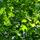Do they know about logarithms yet?

dappled_leaves (15883)“Great Answer” (1) Flag as…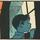Yes, you can solve it algebraically:

2^(x-1)/100 + 0.005 = 5x + 10

Solve for x.

zenvelo (37025)“Great Answer” (1) Flag as…@dappled_leaves I doubt it. I tried using logarithms anyway and couldn’t get it.
@zenvelo Obviously, but I can’t solve the dumbed-down version yet (2^x – 10 = 20). If I can get a hold of that, I was hoping to use the same method on the real problem.

The value of x, by the way, is somewhere between the integers 13 and 14. Guess and check is not a sufficient method for me, either.

dxs (15160)“Great Answer” (0) Flag as…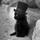Log base 2 of 30 says my husband (maths teacher)
I read him the equation and he said logs would do it
Hope this helps!

Stinley (11520)“Great Answer” (0) Flag as…Solving 2^x – 10 = 20 is pretty easy using logarithms. I think you meant to have an x term in that equation.

I do not see any way of solving an equation with exponential and linear terms in x. You would probably need an approximation method, which would be beyond what the students know.

LostInParadise (29299)“Great Answer” (1) Flag as…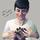2^x – 10 = 20

2^x = 30

x log(2) = log(30)

x = log(30)/log(2) = ~4.9

Mariah (25876)“Great Answer” (1) Flag as…Or did you intend for x-10 to be the exponent?

Mariah (25876)“Great Answer” (0) Flag as…No! I totally messed up the equation! As @LostInParadise suggested, t’s supposed to be 2^x – 10x = 20. Note the x with the 10. The original question was about powers of x and multiples of x. Sorry for the messup.

dxs (15160)“Great Answer” (0) Flag as…If not solvable, I’d be curious to prove it somehow. But that’d involve a pretty clear definition of what it means to compute using algebra. Perhaps its solution does not belong to some algebraically closed field.

Also, the value of x between 13 & 14 is for the more complicated equation: 2^(x-1)/100 + 0.005 = 5x + 10.

dxs (15160)“Great Answer” (0) Flag as…Ah yes. That’s a much harder problem. :P

Wolfram alpha10x%3D+20 doesn’t even give a straightforward solution to that problem and I certainly don’t know how to solve it.

Mariah (25876)“Great Answer” (0) Flag as…Oh, and I also messed up the exponential equation. I put the y-intercept in linear form. It should be just 2^(n-1) / 100. I think…

@Mariah It looks like it solves it by plotting. That’s the only place I see the value (13.96…). I have no clue what that W function is, but it looks like something beyond algebra. I Wikipediaed the function and it’s called the Omega Function. I tried using it based on the Wiki article, but I couldn’t solve it.

dxs (15160)“Great Answer” (0) Flag as…Yeah I’m not familiar with that function either. Bummer :\

Mariah (25876)“Great Answer” (0) Flag as…or